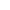Go to content
A histogram is a lot more than a bar chart! In a histogram, the area of the bar, NOT its height, is proportional to frequency. Bars do not have to be of equal width. The height of each bar is called the frequency density.You can get a better display of the maths by downloading special TeX fonts from jsMath. In the meantime, we will do the best we can with the fonts you have, but it may not be pretty and some equations may not be rendered correctly.

## Glossary

### density

the ratio of the mass of an object to its volume

### frequency

Of a function: the rate of repetition of a periodic function.
In statistics: the number of occurances of a value

### frequency density

The frequency density of a frequency distribution is given by
(class frequency)/(class width).

### histogram

A statistical diagram for continuous data similar to a bar chart but where the area of each bar is proportional to the frequency.

### union

The union of two sets A and B is the set containing all the elements of A and B.

Full Glossary List

## This question appears in the following syllabi:

SyllabusModuleSectionTopicExam Year
AQA AS Maths 2017StatisticsData Presentation and InterpretationHistograms-
AQA AS/A2 Maths 2017StatisticsData Presentation and InterpretationHistograms-
AQA GCSE (9-1) Higher (UK)S: StatisticsS3: Grouped Data, Histograms and Cumulative FrequencyHistograms-
CBSE IX (India)StatisticsDisplaying DataHistograms-
CIE IGCSE (9-1) Maths (0626 UK)9 StatisticsB9.4 Graphical RepresentationsHistograms-
Edexcel AS Maths 2017StatisticsData Presentation and InterpretationHistograms-
Edexcel AS/A2 Maths 2017StatisticsData Presentation and InterpretationHistograms-
Edexcel GCSE (9-1) Higher (UK)S: StatisticsS3: Grouped Data, Histograms and Cumulative FrequencyHistograms-
GCSE Higher (UK)StatisticsDisplaying dataHistograms-
OCR AS Maths 2017StatisticsWorking with DataHistograms-
OCR GCSE (9-1) Higher (UK)12: Statistics12.02b: Grouped DataHistograms-
OCR MEI AS Maths 2017StatisticsWorking with DataHistograms-
Universal (all site questions)DDisplaying dataHistograms-